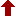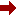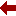#### SLIM21

 Sort Order Chronological Author Title ItemType,Author ItemType,Title Display Format Tabular Publication Bibliography Items / Page 5 10 25

Click the serial number on the left to view the details of the item.
#  AuthorTitleAccn#YearItem Type Claims
1 Timberlake, Todd Keene Classical Mechanics with Maxima I10330 2016 eBook
2 Rejzner, Kasia Perturbative Algebraic Quantum Field Theory I10180 2016 eBook
3 Levi, Decio Symmetries and Integrability of Difference Equations I10153 2017 eBook
4 Tanaka, Akinori Superconformal Index on RP2 ?? S1 and 3D Mirror Symmetry I09758 2016 eBook
5 Landi, Giovanni Linear Algebra and Analytic Geometry for Physical Sciences I09687 2018 eBook
6 Dappiaggi, Claudio Hadamard States from Light-like Hypersurfaces I09659 2017 eBook
7 Konno, Hitoshi Elliptic Quantum Groups I09584 2020 eBook
8 Weingartner, Paul A Alternative Logics. Do Sciences Need Them? I11584 2004 eBook
9 Coecke, Bob Current Research in Operational Quantum Logic I11348 2000 eBook
10 Esposti, Mirko The Mathematical Aspects of Quantum Maps I11187 2003 eBook
 (page:1 / 10) [#98] Next Page Last Page

1.TitleClassical Mechanics with Maxima
Author(s)Timberlake, Todd Keene;Mixon, J. Wilson
PublicationNew York, NY, 1. Imprint: Springer 2. Springer New York, 2016.
DescriptionXI, 258 p. 156 illus : online resource
Abstract NoteThis book guides undergraduate students in the use of Maxima???a computer algebra system???in solving problems in classical mechanics. It functions well as a supplement to a typical classical mechanics textbook. When it comes to problems that are too difficult to solve by hand, computer algebra systems that can perform symbolic mathematical manipulations are a valuable tool. Maxima is particularly attractive in that it is open-source, multiple-platform software that students can download and install free of charge. Lessons learned and capabilities developed using Maxima are easily transferred to other, proprietary software
ISBN,Price9781493932078
Keyword(s)1. ALGEBRA 2. CLASSICAL MECHANICS 3. Computational Mathematics and Numerical Analysis 4. Computer mathematics 5. EBOOK 6. EBOOK - SPRINGER 7. General Algebraic Systems 8. Mathematical Applications in the Physical Sciences 9. Mathematical Methods in Physics 10. MATHEMATICAL PHYSICS 11. MECHANICS 12. PHYSICS
Item TypeeBook
Circulation Data
 Accession# Call# Status Issued To Return Due On Physical Location I10330 On Shelf

2.TitlePerturbative Algebraic Quantum Field Theory : An Introduction for Mathematicians
Author(s)Rejzner, Kasia
PublicationCham, 1. Imprint: Springer 2. Springer International Publishing, 2016.
DescriptionXI, 180 p. 4 illus : online resource
Abstract NotePerturbative Algebraic Quantum Field Theory (pAQFT), the subject of this book, is a complete and mathematically rigorous treatment of perturbative quantum field theory (pQFT) that doesn???t require the use of divergent quantities. We discuss in detail the examples of scalar fields and gauge theories and generalize them to QFT on curved spacetimes. pQFT models describe a wide range of physical phenomena and have remarkable agreement with experimental results. Despite this success, the theory suffers from many conceptual problems. pAQFT is a good candidate to solve many, if not all of these conceptual problems. Chapters 1-3 provide some background in mathematics and physics. Chapter 4 concerns classical theory of the scalar field, which is subsequently quantized in chapters 5 and 6. Chapter 7 covers gauge theory and chapter 8 discusses QFT on curved spacetimes and effective quantum gravity. The book aims to be accessible researchers and graduate students interested in the mathematical foundations of pQFT are the intended audience
ISBN,Price9783319259017
Keyword(s)1. ALGEBRA 2. EBOOK 3. EBOOK - SPRINGER 4. Field theory (Physics) 5. Field Theory and Polynomials 6. MATHEMATICAL PHYSICS 7. Quantum Field Theories, String Theory 8. QUANTUM FIELD THEORY 9. STRING THEORY
Item TypeeBook
Circulation Data
 Accession# Call# Status Issued To Return Due On Physical Location I10180 On Shelf

3.TitleSymmetries and Integrability of Difference Equations : Lecture Notes of the Abecederian School of SIDE 12, Montreal 2016
Author(s)Levi, Decio;Rebelo, Rapha??l;Winternitz, Pavel
PublicationCham, 1. Imprint: Springer 2. Springer International Publishing, 2017.
DescriptionX, 435 p. 67 illus., 26 illus. in color : online resource
Abstract NoteThis book shows how Lie group and integrability techniques, originally developed for differential equations, have been adapted to the case of difference equations. Difference equations are playing an increasingly important role in the natural sciences. Indeed, many phenomena are inherently discrete and thus naturally described by difference equations. More fundamentally, in subatomic physics, space-time may actually be discrete. Differential equations would then just be approximations of more basic discrete ones. Moreover, when using differential equations to analyze continuous processes, it is often necessary to resort to numerical methods. This always involves a discretization of the differential equations involved, thus replacing them by difference ones. Each of the nine peer-reviewed chapters in this volume serves as a self-contained treatment of a topic, containing introductory material as well as the latest research results and exercises. Each chapter is presented by one or more early career researchers in the specific field of their expertise and, in turn, written for early career researchers. As a survey of the current state of the art, this book will serve as a valuable reference and is particularly well suited as an introduction to the field of symmetries and integrability of difference equations. Therefore, the book will be welcomed by advanced undergraduate and graduate students as well as by more advanced researchers
ISBN,Price9783319566665
Keyword(s)1. ALGEBRA 2. Difference and Functional Equations 3. DIFFERENCE EQUATIONS 4. EBOOK 5. EBOOK - SPRINGER 6. Field theory (Physics) 7. Field Theory and Polynomials 8. FUNCTIONAL EQUATIONS 9. Numerical and Computational Physics, Simulation 10. PHYSICS
Item TypeeBook
Circulation Data
 Accession# Call# Status Issued To Return Due On Physical Location I10153 On Shelf

4.TitleSuperconformal Index on RP2 ?? S1 and 3D Mirror Symmetry
Author(s)Tanaka, Akinori
PublicationSingapore, 1. Imprint: Springer 2. Springer Singapore, 2016.
DescriptionXII, 83 p : online resource
Abstract NoteThe author introduces the supersymmetric localization technique, a new approach for computing path integrals in quantum field theory on curved space (time) defined with interacting Lagrangian. The author focuses on a particular quantity called the superconformal index (SCI), which is defined by considering the theories on the product space of two spheres and circles, in order to clarify the validity of so-called three-dimensional mirror symmetry, one of the famous duality proposals. In addition to a review of known results, the author presents a new definition of SCI by considering theories on the product space of real-projective space and circles. In this book, he explains the concept of SCI from the point of view of quantum mechanics and gives localization computations by reducing field theoretical computations to many-body quantum mechanics. He applies his new results of SCI with real-projective space to test three-dimensional mirror symmetry, one of the dualities of quantum field theory. Real-projective space is known to be an unorientable surface like the Mobius strip, and there are many exotic effects resulting from Z2 holonomy of the surface. Thanks to these exotic structures, his results provide completely new evidence of three-dimensional mirror symmetry. The equivalence expected from three-dimensional mirror symmetry is transformed into a conjectural non-trivial mathematical identity through the new SCI, and he performs the proof of the identity using a q-binomial formula
ISBN,Price9789811013980
Keyword(s)1. ALGEBRA 2. EBOOK 3. EBOOK - SPRINGER 4. Field theory (Physics) 5. Field Theory and Polynomials 6. Mathematical Applications in the Physical Sciences 7. MATHEMATICAL PHYSICS 8. Quantum Field Theories, String Theory 9. QUANTUM FIELD THEORY 10. STRING THEORY
Item TypeeBook
Circulation Data
 Accession# Call# Status Issued To Return Due On Physical Location I09758 On Shelf

5.TitleLinear Algebra and Analytic Geometry for Physical Sciences
Author(s)Landi, Giovanni;Zampini, Alessandro
PublicationCham, 1. Imprint: Springer 2. Springer International Publishing, 2018.
DescriptionXII, 345 p : online resource
Abstract NoteA self-contained introduction to finite dimensional vector spaces, matrices, systems of linear equations, spectral analysis on euclidean and hermitian spaces, affine euclidean geometry, quadratic forms and conic sections. The mathematical formalism is motivated and introduced by problems from physics, notably mechanics (including celestial) and electro-magnetism, with more than two hundreds examples and solved exercises. Topics include: The group of orthogonal transformations on euclidean spaces, in particular rotations, with Euler angles and angular velocity. The rigid body with its inertia matrix. The unitary group. Lie algebras and exponential map. The Dirac???s bra-ket formalism. Spectral theory for self-adjoint endomorphisms on euclidean and hermitian spaces. The Minkowski spacetime from special relativity and the Maxwell equations. Conic sections with the use of eccentricity and Keplerian motions. An appendix collects basic algebraic notions like group, ring and field; and complex numbers and integers modulo a prime number. The book will be useful to students taking a physics or engineer degree for a basic education as well as for students who wish to be competent in the subject and who may want to pursue a post-graduate qualification
ISBN,Price9783319783611
Keyword(s)1. ALGEBRA 2. APPLIED MATHEMATICS 3. Computer science???Mathematics 4. EBOOK 5. EBOOK - SPRINGER 6. ENGINEERING MATHEMATICS 7. GEOMETRY 8. Linear and Multilinear Algebras, Matrix Theory 9. Math Applications in Computer Science 10. Mathematical and Computational Engineering 11. Mathematical Applications in the Physical Sciences 12. Mathematical Methods in Physics 13. MATHEMATICAL PHYSICS 14. MATRIX THEORY 15. PHYSICS
Item TypeeBook
Circulation Data
 Accession# Call# Status Issued To Return Due On Physical Location I09687 On Shelf

6.Author(s)Dappiaggi, Claudio;Moretti, Valter;Pinamonti, Nicola
PublicationCham, 1. Imprint: Springer 2. Springer International Publishing, 2017.
DescriptionVIII, 106 p. 2 illus : online resource
Abstract NoteThis book provides a rather self-contained survey of the construction of Hadamard states for scalar field theories in a large class of notable spacetimes, possessing a (conformal) light-like boundary. The first two sections focus on explaining a few introductory aspects of this topic and on providing the relevant geometric background material. The notions of asymptotically flat spacetimes and of expanding universes with a cosmological horizon are analysed in detail, devoting special attention to the characterization of asymptotic symmetries. In the central part of the book, the quantization of a real scalar field theory on such class of backgrounds is discussed within the framework of algebraic quantum field theory. Subsequently it is explained how it is possible to encode the information of the observables of the theory in a second, ancillary counterpart, which is built directly on the conformal (null) boundary. This procedure, dubbed bulk-to-boundary correspondence, has the net advantage of allowing the identification of a distinguished state for the theory on the boundary, which admits a counterpart in the bulk spacetime which is automatically of Hadamard form. In the last part of the book, some applications of these states are discussed, in particular the construction of the algebra of Wick polynomials. This book is aimed mainly, but not exclusively, at a readership with interest in the mathematical formulation of quantum field theory on curved backgrounds
ISBN,Price9783319643434
Keyword(s)1. ALGEBRA 2. COSMOLOGY 3. EBOOK 4. EBOOK - SPRINGER 5. Field theory (Physics) 6. Field Theory and Polynomials 7. MATHEMATICAL PHYSICS 8. Quantum Field Theories, String Theory 9. QUANTUM FIELD THEORY 10. STRING THEORY
Item TypeeBook
Circulation Data
 Accession# Call# Status Issued To Return Due On Physical Location I09659 On Shelf

7.TitleElliptic Quantum Groups : Representations and Related Geometry
Author(s)Konno, Hitoshi
PublicationSingapore, 1. Imprint: Springer 2. Springer Singapore, 2020.
DescriptionXIII, 131 p. 3 illus : online resource
Abstract NoteThis is the first book on elliptic quantum groups, i.e., quantum groups associated to elliptic solutions of the Yang-Baxter equation. Based on research by the author and his collaborators, the book presents a comprehensive survey on the subject including a brief history of formulations and applications, a detailed formulation of the elliptic quantum group in the Drinfeld realization, explicit construction of both finite and infinite-dimensional representations, and a construction of the vertex operators as intertwining operators of these representations. The vertex operators are important objects in representation theory of quantum groups. In this book, they are used to derive the elliptic q-KZ equations and their elliptic hypergeometric integral solutions. In particular, the so-called elliptic weight functions appear in such solutions. The author???s recent study showed that these elliptic weight functions are identified with Okounkov???s elliptic stable envelopes for certain equivariant elliptic cohomology and play an important role to construct geometric representations of elliptic quantum groups. Okounkov???s geometric approach to quantum integrable systems is a rapidly growing topic in mathematical physics related to the Bethe ansatz, the Alday-Gaiotto-Tachikawa correspondence between 4D SUSY gauge theories and the CFT???s, and the Nekrasov-Shatashvili correspondences between quantum integrable systems and quantum cohomology. To invite the reader to such topics is one of the aims of this book
ISBN,Price9789811573873
Keyword(s)1. ALGEBRA 2. EBOOK 3. EBOOK - SPRINGER 4. GROUP THEORY 5. Group Theory and Generalizations 6. Mathematical Applications in the Physical Sciences 7. MATHEMATICAL PHYSICS 8. Order, Lattices, Ordered Algebraic Structures 9. Ordered algebraic structures
Item TypeeBook
Circulation Data
 Accession# Call# Status Issued To Return Due On Physical Location I09584 On Shelf

8.TitleAlternative Logics. Do Sciences Need Them?
Author(s)Weingartner, Paul A
PublicationBerlin, Heidelberg, 1. Imprint: Springer 2. Springer Berlin Heidelberg, 2004.
DescriptionXVII, 368 p : online resource
Abstract NoteInitially proposed as rivals of classical logic, alternative logics have become increasingly important in sciences such as quantum physics, computer science, and artificial intelligence. The contributions collected in this volume address and explore the question whether the usage of logic in the sciences, especially in modern physics, requires a deviation from classical mathematical logic. The articles in the first part of the book set the scene by describing the context and the dilemma when applying logic in science. In Part II the authors offer several logics that deviate in different ways from classical logics. The twelve papers in Part III investigate in detail specific aspects such as quantum logic, quantum computation, computer-science considerations, praxic logic, and quantum probability. Most of the contributions are revised and partially extended versions of papers presented at a conference of the same title of the Acad??mie Internationale de Philosophie des Sciences held at the Internationales Forschungszentrum Salzburg in May 1999. Others have been added to complete the picture of recent research in alternative logics as they have been developed for applications in the sciences
ISBN,Price9783662056790
Keyword(s)1. ALGEBRA 2. COMPUTERS 3. EBOOK 4. EBOOK - SPRINGER 5. Order, Lattices, Ordered Algebraic Structures 6. Ordered algebraic structures 7. PHILOSOPHY 8. Philosophy, general 9. QUANTUM COMPUTERS 10. Quantum Information Technology, Spintronics 11. QUANTUM PHYSICS 12. SPINTRONICS 13. Theory of Computation
Item TypeeBook
Circulation Data
 Accession# Call# Status Issued To Return Due On Physical Location I11584 On Shelf

9.TitleCurrent Research in Operational Quantum Logic : Algebras, Categories, Languages
Author(s)Coecke, Bob;Moore, David;Wilce, Alexander
PublicationDordrecht, 1. Imprint: Springer 2. Springer Netherlands, 2000.
DescriptionVII, 325 p : online resource
Abstract NoteThe present volume has its origins in a pair of informal workshops held at the Free University of Brussels, in June of 1998 and May of 1999, named "Current Research 1 in Operational Quantum Logic". These brought together mathematicians and physicists working in operational quantum logic and related areas, as well as a number of interested philosophers of science, for a rare opportunity to discuss recent developments in this field. After some discussion, it was decided that, rather than producing a volume of conference proceedings, we would try to organize the conferees to produce a set of comprehensive survey papers, which would not only report on recent developments in quantum logic, but also provide a tutorial overview of the subject suitable for an interested non-specialist audience. The resulting volume provides an overview of the concepts and methods used in current research in quantum logic, viewed both as a branch of mathemati?? cal physics and as an area of pure mathematics. The first half of the book is concerned with the algebraic side of the subject, and in particular the theory of orthomodular lattices and posets, effect algebras, etc. In the second half of the book, special attention is given to categorical methods and to connections with theoretical computer science. At the 1999 workshop, we were fortunate to hear three excellent lectures by David J. Foulis, represented here by two contributions. Dave's work, spanning 40 years, has helped to define, and continues to reshape, the field of quantum logic
ISBN,Price9789401712019
Keyword(s)1. ALGEBRA 2. Applications of Mathematics 3. APPLIED MATHEMATICS 4. Category theory (Mathematics) 5. Category Theory, Homological Algebra 6. EBOOK 7. EBOOK - SPRINGER 8. ENGINEERING MATHEMATICS 9. GROUP THEORY 10. Group Theory and Generalizations 11. Homological algebra 12. Order, Lattices, Ordered Algebraic Structures 13. Ordered algebraic structures 14. QUANTUM PHYSICS
Item TypeeBook
Circulation Data
 Accession# Call# Status Issued To Return Due On Physical Location I11348 On Shelf

10.TitleThe Mathematical Aspects of Quantum Maps
Author(s)Esposti, Mirko;Graffi, Sandro
PublicationBerlin, Heidelberg, 1. Imprint: Springer 2. Springer Berlin Heidelberg, 2003.
DescriptionXI, 171 p : online resource
Abstract NoteQuantum maps are presented with special emphasis on their physical origin. They represent a testing ground for understanding concepts in quantized chaotic systems. The book develops and teaches the modern mathematical methods from analytic and algebraic number theory as applied to quantum maps. It gives a broad and in-depth overview of the mathematical problems arising in this area. Also treated are the numerical aspects in quantum chaos such as eigenvalue and eigenfunctions computations for chaotic quantum systems. The book addresses scientists and advanced students in mathematics and mathematical physics
ISBN,Price9783540370451
Keyword(s)1. ALGEBRA 2. COMPLEX SYSTEMS 3. Computational Mathematics and Numerical Analysis 4. Computer mathematics 5. DYNAMICAL SYSTEMS 6. EBOOK 7. EBOOK - SPRINGER 8. Mathematical Methods in Physics 9. MATHEMATICAL PHYSICS 10. PHYSICS 11. QUANTUM PHYSICS 12. STATISTICAL PHYSICS 13. Theoretical, Mathematical and Computational Physics
Item TypeeBook